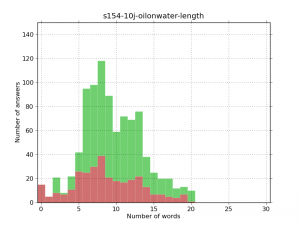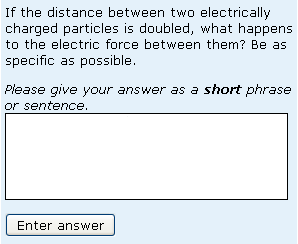# More on length of student answers

So what else affects the length of student responses to short-answer free-text questions? I’ve already alluded to the fact that formative responses are shorter than summative ones and to the fact that different questions lead to responses of wildly varying length. The two figures below show the length distributions for two more questions, both from uses with the length filter and simple warning ‘You should give your answer as a short phrase or sentence.’ The questions themselves are shown in Figures 3 and 4 at the end of this posting.Figure 1 Distribution of length of 927 responses to the Oil on water question.Figure 2 Distribution of length of 1187 responses to the Electric force question.

Look first at the shape of the overall envelope of the distributions. The first thing to notice is that Figure 1 is a broad distribution with a maximum at 8 words, whilst Figure 2 has distinct peaks at 9 and 20 words ( in contrast to the Snowflake question, the peak at 20 words for the Electric force question is an artefact of the question itself; it was there when students were not given any guidance as to the length of their answer and when they were told that their answer should be no more than 20 words).

Next, compare the parts of the graphs coloured red and green. Green represents correct responses; red represents incorrect responses. It turns out that for Oil on water, the distribution of lengths is similar whether responses are correct or incorrect. However, for Electric force, the two peaks are there for correct responses but not for incorrect.

How can we explain these things? It turns out that for Electric force, the peaks correspond to characteristic types of correct response. Responses are not necessarily worded in exactly the same way, but the peak on 9 words corresponds to responses that precisely state the effect of moving the two charges apart, whist the peak on 20 words corresponds to responses that precisely state the effect of moving the two charges and also say why the effect is as it is e.g. The force decreases by a factor of four  since distance and electric force are related by an inverse square law. Such responses are usually correct so the peaks are simply not there for incorrect responses. For the Oil on water question, neither correct nor incorrect responses can be so easily classified. There are many different correct and incorrect responses; they happen to have an ‘average’ length of 8 words.

Another question to ask in the context of length is whether responses get longer or shorter at second and third attempt. There are fewer responses by second and third attempt (students who have already got the question right are no longer attempting it) but there is some evidence that responses tend to get slightly longer i.e. that some students are adding to their first attempt response at the second attempt and/or adding to their second attempt response at the third attempt. I will explore this further in a later posting.

The questions whose responses are discussed here are shown below:Figure 3 The Oil on water questionFigure 4 The Electric force question

This entry was posted in short-answer free text questions, student engagement and tagged , , . Bookmark the permalink.